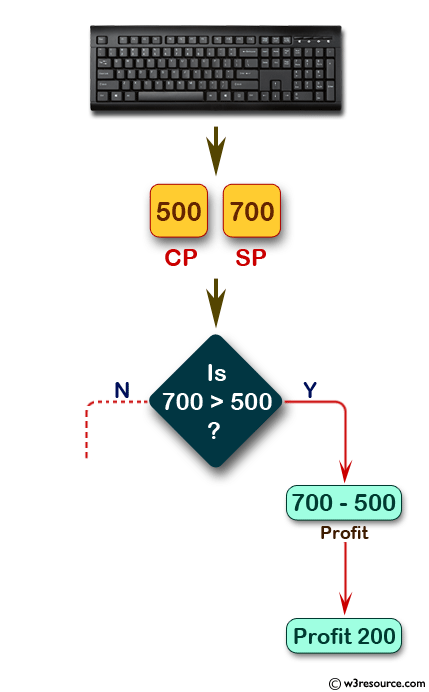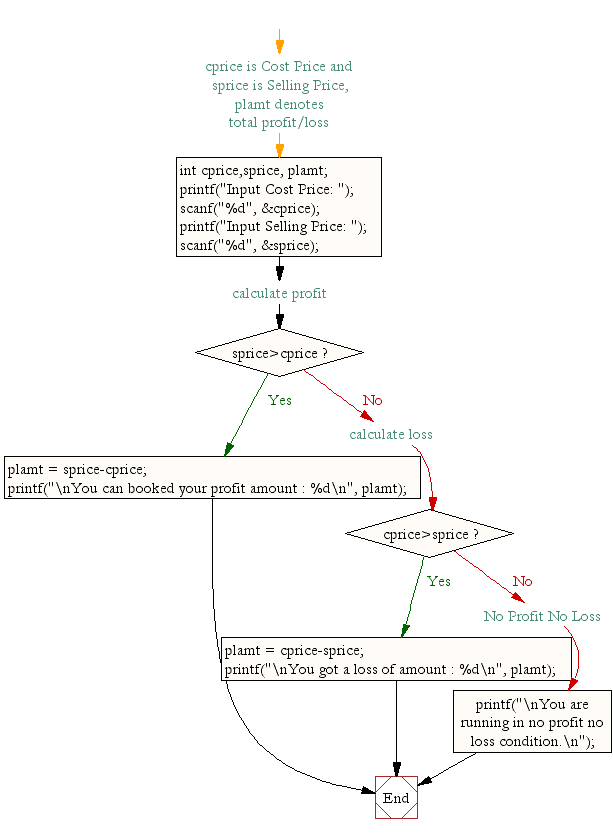﻿ C Program: Calculate profit and loss - w3resource# C Exercises: Calculate profit and loss

## C Conditional Statement: Exercise-18 with Solution

Write a C program to calculate profit and loss on a transaction.

Pictorial Presentation:Sample Solution:

C Code:

``````#include <stdio.h>
void main()
{
int cprice,sprice, plamt; //cprice is Cost Price and sprice is Selling Price, plamt denotes total profit/loss

printf("Input Cost Price: ");
scanf("%d", &cprice);
printf("Input Selling Price: ");
scanf("%d", &sprice);

if(sprice>cprice) //calculate profit
{
plamt = sprice-cprice;
printf("\nYou can booked your profit amount : %d\n", plamt);
}
else if(cprice>sprice) //calculate loss
{
plamt = cprice-sprice;
printf("\nYou got a loss of amount : %d\n", plamt);
}
else //No Profit No Loss
{
printf("\nYou are running in no profit no loss condition.\n");
}
}
```
```

Sample Output:

```Input Cost Price: 500
Input Selling Price: 700

You can booked your profit amount : 200
```

Flowchart:C Programming Code Editor:

Improve this sample solution and post your code through Disqus.

What is the difficulty level of this exercise?

Test your Programming skills with w3resource's quiz.

﻿

## C Programming: Tips of the Day

Why do C and C++ compilers allow array lengths in function signatures when they're never enforced?

It is a quirk of the syntax for passing arrays to functions.

Actually it is not possible to pass an array in C. If you write syntax that looks like it should pass the array, what actually happens is that a pointer to the first element of the array is passed instead.

Since the pointer does not include any length information, the contents of your [] in the function formal parameter list are actually ignored.

Ref : https://bit.ly/3fhlvdH